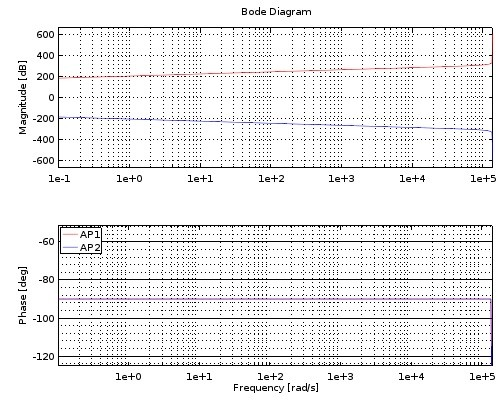## Correct transfer function with 1st order IIR filterStarted by 6 years ago2 replieslatest reply 6 years ago163 views

What diffreneces it makes in magnitude and/or phase response when using

H(z) = (b0 + b1*z^-1 + b2*z^-2)/(a0 + a1*z^-1 + a2*z^-2) by adding b2=0, a2=0

H(z) = (b0 + b1*z^-1)/(a0 + a1*z^-1)

for 1st order filter?

All software I'm using for to build VST plug-ins (SynthEdit, FlowStone, etc.) implements a biquad (BLT) module for coefficient input and now it looks like by using it I can't get 90° phase response with a filter designed using Octave (by Octave plot phase is 90°). I have tried with single filter, series and parallel connections. When two 1st order filters (given below) are connected in serie it shows 0dB/180° and with parallel connection ~+303.5dB/0°.

Here are the Octave plots (both filters has the same phase response):and filter coefficients I'm playing with:

AP1 = [ -1.50119987579016e+015 1.50119987579016e+015 1.0 0.999999999999999 ]
AP2 = [  6.66133814775094e-016 6.66133814775094e-016 1.000000000000001 -1.0 ]

EDIT: Ok, digged the web a bit more and found these three (1 2 3) articles published at analog.com.

EDIT2: Here are responses SynthEdit gives (I have the other filter in Left cahennel and the other in Right channel of plug-in):

MagnitudePhasePhase invertedAs seen in plots, something happens for the magnitudes and phases when filters are implemented as VST plug-in?

[ - ]The coefficients you are using are on the extreme limits of the possibilities of double precision floating point arithmetics.

On double precision you have 53 bits on the mantissa and 11 bits on the exponent. Using these coefficients you may have difficulties to keep the needed precision after addition/subtraction.

[ - ]